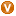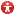AnyLogic

# Variable

Agent can contain variables. Variables are generally used to store the results of model simulation or to model some data units or object characteristics, changing over time. AnyLogic supports two types of variables — variables and collections.

Collections are used for defining data objects that group multiple elements into a single unit.

Variable is a simple variable of an arbitrary scalar type or Java class. It always has some value assigned. You specify the variable’s initial value in the Initial value property of the variable. If an initial value is not specified, Java rules apply, for example a variable of type double is set to 0.

The variable’s value can be changed during the model simulation using the assignment operator, e.g. you can set integer variable myVariable to be equal to 5 by the following code:

myVariable = 5;

However, you cannot set any formula for a variable. If you need a variable that changes its value according to specified differential equation, please use Stock. Otherwise, if you need a variable that changes its value according to some algebraic formula, please use dynamic variables. You can find both these variables on the System Dynamics palette.

You can declare Java variables in the Additional class code field in the Advanced Java properties section of the agent type. Variables declared in the code can also be accessed within this object, but defining them visually using variables is much more efficient.

## Types of variables

Alike other simulation tools AnyLogic supports variables of primitive types: double, integer, boolean. But only AnyLogic gives you infinite possibilities in defining data units by supporting variables of any Java classes.

You can define variables of common Java classes — e.g., a variable of String class to store character strings, as well as of your own classes, defined elsewhere in your model.

### Creating a variable

To create a variable

1. Drag theVariable element from theAgent palette to the agent diagram.
2. In the Properties view, type the name of the variable in the Name edit box. The name is used to identify and access the variable from code.
3. Specify the type of the variable. You can choose one of the most-used types (int, double, boolean, String) using the corresponding option from the Type buttons group. However, if you need to define a variable of some other Java class, choose Other option and type the required class name in the edit box to the right.
4. Specify the variable’s initial value in the Initial value edit box. The initial value of the variable can be changed afterwards during the model simulation. If an initial value is not specified, Java rules apply, for example a variable of type double is set to 0.
5. Finally, if you want to make this variable a constant and prevent it from modifying at model runtime, open the Advanced properties section and select the Constant checkbox. If you set this variable as Constant, you cannot use parameters, stocks, flows, and dynamic variables in the expression specified in the Initial value field.

## Properties

General

Name — The name of the variable. The name is used to identify and access the variable from code.

Show name — If selected, the name of the variable is displayed on a presentation diagram.

Ignore — If selected, the variable is excluded from the model.

Visible — If selected, the variable is visible on a presentation at runtime.

Type — The type of the variable. Choose, whether you want variable of some most-used type (int, double, boolean, String), or of some Other Java class using the controls to the right.

Initial value — You can define the initial value for the variable here. The initial value of the variable can be changed afterwards during the model simulation. If an initial value is not specified, Java rules apply, for example a variable of type double is set to 0.
If you set this variable as Constant, you cannot use parameters, stocks, flows, and dynamic variables in the expression specified in the Initial value field. In this case you can use only values and other constants here.

Access — The access type of the variable. There are four access types:

• public — the variable can be accessed from anywhere.
• private — the variable can be accessed from this agent class only.
• protected — the variable can be accessed from this agent class and its subclasses.
• default — the variable can be accessed anywhere within this model.

Static — [Only visible if Library developer mode is enabled] If selected, the variable is static, i.e. it will have the same value for all instances of this class in the model (e.g. for all agents inside the population).

Do not use static variables, if you plan to run iterations of complex experiments (optimization, parameter variation, etc.) in parallel on different processor cores (this is set by the experiment’s advanced option Allow parallel evaluations).
Static variables are not saved into a snapshot file when you save/restore a state of a running model. If you do not alter the value of a static variable in your model, the state of the restored model will be exactly the same as you saved it into the snapshot. However, if you somehow change the value of a static variable (e.g. in agent’s On startup code), the modified value won’t be saved into a snapshot, and the restored state of the model will differ from the state you have previously saved.

Constant — If selected, the variable is made constant, i.e. it has the same value at all times and can not be changed during the simulation. Making variable a constant, you prevent it from unwanted modifying.

Save in snapshot — [Enabled if Static checkbox is not selected] If selected, the variable will be saved in the model snapshot.

System dynamics units — If selected, you will be able to specify units of measurements for this variable in the edit box below. Having specified units for elements of your model, you may perform unit checking to find out dimension inconsistencies in the model.